Documentation

## Geometric Transformation Types for Control Point Registration

The Image Processing Toolbox™ provides functionality for applying geometric transformations to register images.

For control point registration, the `fitgeotrans` function can infer the parameters for the following types of transformations, listed in order of complexity.

Transformation Type

DescriptionMinimum Number of Control Point PairsExample
`'nonreflective similarity'`Use this transformation when shapes in the moving image are unchanged, but the image is distorted by some combination of translation, rotation, and scaling. Straight lines remain straight, and parallel lines are still parallel. 2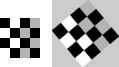`'similarity'`Same as `'nonreflective similarity'` with the addition of optional reflection.3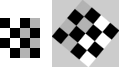`'affine'`Use this transformation when shapes in the moving image exhibit shearing. Straight lines remain straight, and parallel lines remain parallel, but rectangles become parallelograms.3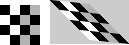`'projective'`Use this transformation when the scene appears tilted. Straight lines remain straight, but parallel lines converge toward a vanishing point.4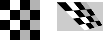`'polynomial'`Use this transformation when objects in the image are curved. The higher the order of the polynomial, the better the fit, but the result can contain more curves than the fixed image.

6 (order 2)

10 (order 3)

15 (order 4)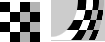`'piecewise linear'`Use this transformation when parts of the image appear distorted differently.4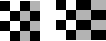`'lwm'`Use this transformation (local weighted mean), when the distortion varies locally and piecewise linear is not sufficient. 6 (12 recommended)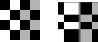The first four transformations, `'nonreflective similarity'`, `'affine'`, `'projective'`, and `'polynomial'` are global transformations. In these transformations, a single mathematical expression applies to an entire image. The last two transformations, `'piecewise linear'` and `'lwm'` (local weighted mean), are local transformations. In these transformations, different mathematical expressions apply to different regions within an image. When exploring how different transformations affect the images you are working with, try the global transformations first. If these transformations are not satisfactory, try the local transformations: the piecewise linear transformation first, and then the local weighted mean transformation.

Your choice of transformation type affects the number of control point pairs you must select. For example, a nonreflective similarity transformation requires at least two control point pairs. A fourth order polynomial transformation requires 15 control point pairs. For more information about these transformation types, and the special syntaxes they require, see `cpselect`.